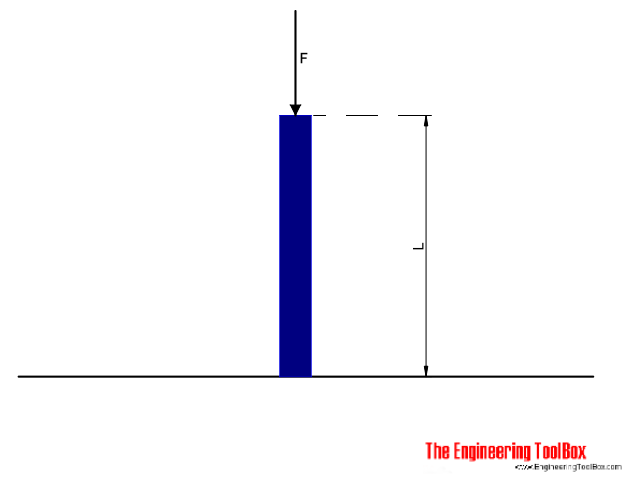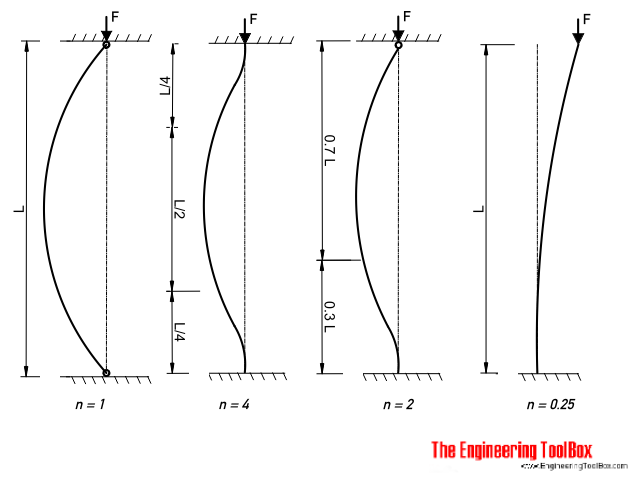Engineering ToolBox - Resources, Tools and Basic Information for Engineering and Design of Technical Applications!

# Euler's Column Formula

## Calculate buckling of columns.Columns fail by buckling when their critical load is reached. Long columns can be analysed with the Euler column formula

F = n π2 E I / L2                             (1)

where

F = allowable load (lb, N)

n = factor accounting for the end conditions

E = modulus of elastisity (lb/in2, Pa (N/m2))

L = length of column (in, m)

I = Moment of inertia (in4, m4)

### Factor Counting for End Conditions• column pivoted in both ends : n = 1
• both ends fixed : n = 4
• one end fixed, the other end rounded : n = 2
• one end fixed, one end free : n = 0.25

### Note!

Equation (1) is sometimes expressed with a k factor accounting for the end conditions:

F = π2 E I / (k L)2                             (1b)

where

k = (1 / n)1/2    factor accounting for the end conditions

 n 1 4 2 0.25 k 1 0.5 0.7 2

### Example - A Column Fixed in both Ends

An column with length 5 m is fixed in both ends. The column is made of an Aluminium I-beam 7 x 4 1/2 x 5.80 with a Moment of Inertia iy = 5.78 in4. The Modulus of Elasticity of aluminum is 69 GPa (69 109 Pa) and the factor for a column fixed in both ends is 4.

The Moment of Inertia can be converted to metric units like

Iy = 5.78 in4 (0.0254 m/in)4

= 241 10-8  m4

The Euler buckling load can then be calculated as

F = (4) π2 (69 109 Pa) (241 10-8  m4) / (5 m)2

=  262594 N

= 263 kN

### Slenderness Ratio

The term "L/r" is known as the slenderness ratio. L is the length of the column and r is the radiation of gyration for the column.

• higher slenderness ratio - lower critical stress to cause buckling
• lower slenderness ratio - higher critical stress to cause buckling
• slenderness ratios L/r < 40: "short columns" where failure mode is crushing (yielding)
• slenderness ratios 40 < L/r < 120: "intermediate columns" where failure mode is a combination of crushing (yielding) and buckling
• slenderness ratio of 120 < L/r < 200: "long columns" where failure mode is buckling

## Related Topics

• Beams and Columns - Deflection and stress, moment of inertia, section modulus and technical information of beams and columns.

## Engineering ToolBox - SketchUp Extension - Online 3D modeling!

Add standard and customized parametric components - like flange beams, lumbers, piping, stairs and more - to your Sketchup model with the Engineering ToolBox - SketchUp Extension - enabled for use with the amazing, fun and free SketchUp Make and SketchUp Pro .Add the Engineering ToolBox extension to your SketchUp from the SketchUp Pro Sketchup Extension Warehouse!

Translate

## Privacy

We don't collect information from our users. Only emails and answers are saved in our archive. Cookies are only used in the browser to improve user experience.

Some of our calculators and applications let you save application data to your local computer. These applications will - due to browser restrictions - send data between your browser and our server. We don't save this data.

## Citation

• Engineering ToolBox, (2012). Euler's Column Formula. [online] Available at: https://www.engineeringtoolbox.com/euler-column-formula-d_1813.html [Accessed Day Mo. Year].

Modify access date.

. .

#### Scientific Online Calculator6 24